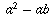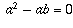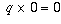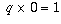Navigation Panel:Go backward to This is Not the FallacyGo up to 1=2: A Proof using Beginning AlgebraSwitch to text-only version (no graphics)Go to University of Toronto Mathematics Network Home Page

# Congratulations; you have correctly identified the fallacious step!

To "cancel" a quantity from both sides of an equation is to divide both sides of the equation by it. So, in this step of the proof we are attempting to divide both sides of the equation by.

However, division only makes sense when the number you are dividing by is non-zero. In this proof,, because we assumed in step 1 that a=b!

Therefore, it is not legitimate to divide both sides of the equation by, because that would be division by zero, which does not make any sense (as explained below).

In essence, this proof boils down to saying "1 times 0 equals 2 times 0, therefore 1 equals 2". The fallacy is that, just because two numbers give you the same answer (zero) after you multiply them each by zero, doesn't necessarily mean that the two numbers are the same, because anything when multiplied by zero gives zero.

This is also the reason division by zero does not make sense: there isn't just one unambiguously determined number q such that, so there isn't any number that we can uniquely and unambiguously define the quotient 0/0 to be.

If you tried to divide 1 (or some other non-zero number) by 0, you'd run into a different problem: in this case, there is no number q at all such that, so there is nothing that we can define the quotient 1/0 to be.

That's why division by zero is undefined (not just because it's a rule somebody decided on!)

To see the explanations for the other steps, finding out exactly why they're correct (or, in some cases, finding out which other steps have slight mistakes in them), go back to the list of steps in the proof. To try your hand at finding the fallacy in a different problem, go back to the Classic Fallacies index page.
Navigation Panel: